Go to: Related nodes. Attributes.

The Multiply Divide utility node allows you to multiply or divide input attributes. It has three parts --- two input attributes, an operator that you apply to the attributes, and an output attribute that holds the result of the operation.

All the input and output attributes have x, y, and z components; that way you can use this node to multiply colors or other triple-value things. If you just want to work with single values, then just use one of the x, y, or z channels.

Note: This node does not do proper vector mathematics, such as dot products and cross products. To do those kind of calculations, use the Vector Product utility node.

There are four settings for the operator; Do nothing, Multiply, Divide, and Power. They are explained below.

Let's say you have set the operator to Multiply. Then this node does the following calculations:

outputX = input1X * input2X
outputY = input1Y * input2Y
outputZ = input1Z * input2Z

In the table below, important attributes have their names listed in bold in the description column.

This node is MP safe

Node nameParentsClassificationMFn typeCompatible function sets
multiplyDividedependNode
utility/generalkMultiplyDividekBase
kNamedObject
kDependencyNode
kMultiplyDivide

## Attributes (13)

Long name (short name)TypeDefaultFlags
`operation` (`op`) enum1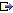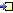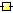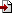Operation controls the operation performed by this node. The settings are: No operation: Output is set to equal Input 1. Input 2 is completely ignored. Multiply: Output is set to equal Input 1 times Input 2. Divide: Output is set to equal Input 1 divided by Input 2. Power: Output is set to equal Input 1 raised to the power of Input 2. Tip: To calculate the square root of Input 1, set Operation to Power, and set Input 2 to 0.5
`input1` (`i1`) float30.0, 0.0, 0.0Input 1: The first input value
`input1X` (`i1x`) float0.0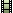The x component of Input 1
`input1Y` (`i1y`) float0.0The y component of Input 1
`input1Z` (`i1z`) float0.0The z component of Input 1
`input2` (`i2`) float31.0, 1.0, 1.0Input 2:The second input value
`input2X` (`i2x`) float0.0The x component of Input 2
`input2Y` (`i2y`) float0.0The y component of Input 2
`input2Z` (`i2z`) float0.0The z component of Input 2
`output` (`o`) float30.0, 0.0, 0.0Output is the values calculated by this node.
`outputX` (`ox`) float0.0The X component of Output
`outputY` (`oy`) float0.0The Y component of Output
`outputZ` (`oz`) float0.0The Z component of Output